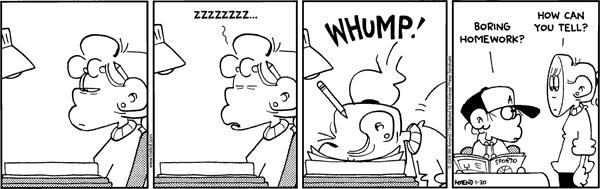Due to the nature of the exercises in this homework, instead of the usual 0 - 1 - 2 grading for each exercise, we will instead utilize 0 - 0.5 - 1 grading for each exercise part.

# Exercise 1

Before it closed, Ron Swanson was a frequent patron of Charles Mulligan’s Steakhouse in Indianapolis, Indiana. Ron enjoyed the experience so much, during each visit he took a picture with his steak.Ron also weighed each steak he consumed. He has a record of eating six “22 ounce” Charles Mulligan’s porterhouse steaks. Ron found that these six steaks weighed

$22.4 \text{ oz}, \ 20.8 \text{ oz}, \ 21.6 \text{ oz}, \ 20.2 \text{ oz}, \ 21.4 \text{ oz}, \ 22.0 \text{ oz}$

Suppose that the weight of “22 ounce” Charles Mulligan’s porterhouse steaks follow a $$N(\mu, \sigma^2)$$ distribution and that Ron’s six steaks were a random sample.

(a) Compute the sample standard deviation, $$s$$, of these six steaks.

(b) Construct a $$95\%$$ two-sided confidence interval for the true mean weight of a “22 ounce” Charles Mulligan’s porterhouse steak, $$\mu$$.

(c) Construct a $$95\%$$ confidence lower bound for the true mean weight of a “22 ounce” Charles Mulligan’s porterhouse steak, $$\mu$$.

(d) Construct a $$90\%$$ two-sided confidence interval for the true standard deviation of the weight of a “22 ounce” Charles Mulligan’s porterhouse steak, $$\sigma$$.

# Exercise 2

The 2020 United States presidential election is beginning to receive a lot of media coverage now that many Democrats have announced their intentions to run in their primary. As in previous Democratic primaries, healthcare is expected to be an issue that receives a lot of attention. Positions on this issue range from revamping the Affordable Care Act, to creating a single-payer health care system, possibly through the expansion of the current Medicare system to all Americans.

A recent survey, conducted by Hill.TV and the HarrisX polling company, asked the question:

“Would you support or oppose Medicare to every American?”

This survey was conducted online within the United States from October 19 - 20, 2018 among 1000 registered voters. The results reflect a nationally representative sample of registered voters. (Results were weighted for age within gender, region, race/ethnicity, income, political party, and education where necessary to align them with their actual proportions in the population. For the purpose of this assignment, assume independence.)

Of the 1000 individuals surveyed, 700 said that they support Medicare for All.

(a) Construct a $$90\%$$ confidence interval for $$p$$, the true proportion of registered voters that support Medicare for All.

(b) Of those surveyed, only 20 were from Generation Z, the youngest block of voters. Suppose that another pollster wants to estimate the true proportion of Generation Z that supports Medicare for All to within 0.05, with $$99\%$$ confidence. How many individuals from Generation Z should this pollster poll? Assume the pollster has no prior knowledge about the proportion.

(c) Now assume that pollster believes that support for Medicare for All within Generation Z is somewhere between $$60\%$$ and $$90\%$$ and they would like to estimate the true proportion of Generation Z that supports Medicare for All to within 0.05, with $$95\%$$ confidence. How many individuals from Generation Z should this pollster poll?

(d) In the original poll, 370 Democratic voters were sampled, of which, 319 support Medicare for All. Of the 320 Republican voters surveyed, 166 support Medicare for All. Let $$p_D$$ be the true proportion of Democratic voters who support Medicare for All and let $$p_R$$ be the true proportion of Republican voters who support Medicare for All. Calculate a 99% confidence interval for $$p_D - p_R$$.

# Exercise 3

Suppose students in a Statistics class are interested in the average score of an exam, but the instructor has only graded (a random sample of) 13 of the (many) exams. The instructor states that a $$90\%$$ confidence interval for the true mean is given by $$(79.14, 82.86)$$ and that you can assume the grades follow a normal distribution.

Using only this information, calculate $$\bar{x}$$, $$s$$, and finally, a $$95\%$$ confidence interval for $$\mu$$, the true mean of the exam.

# Exercise 4

Professor Professorson, a researcher at Greendale Community College, is interested in the effect of caffeine on the typing speed of students. Professorson obtains a random sample of 8 students who are given 400 mg of caffeine then given a typing test. (Don’t try this at home.) They type an average of 51.4 words per minute (wpm), with a sample standard deviation of 12.3 wpm. He also obtains a random sample of 13 students who are given a placebo before the typing test. The placebo group types an average of 43.9 wpm, with a sample standard deviation of 15.1 wpm. Assume typing speeds follow a normal distribution in both groups.

Construct a 99% confidence interval for $$\mu_C - \mu_P$$, the true difference in average typing speed between the caffeine and placebo groups. Assume that the two population variances are equal.